﻿ 3.7 Forward Interest Ratesoffice (412) 967-9367
fax (412) 967-5958
toll-free 1 (800) 214-3480

3.7 Forward Interest Rates

A yield curve embodies information about implied interest rates over future periods of time. These implied future interest rates are referred to as forward interest rates. For example, the overlap between the spot one year interest rate and the spot two year interest rate implies an interest rate for the period of time between Year 1 and Year 2. This interest rate is referred to as the one year forward interest rate, starting at the end of Year 1. This forward interest rate is calculated from the two spot rates, as the following discussion illustrates.

In order to keep things as clear as possible, let the symbol trT (the left subscript is t and the right subscript T) denote the yield to maturity in-period t of a zero-coupon bond maturing at Period T. The current period is period zero. Thus, if t = 0 and T is the maturity date, this is the spot interest rate on a zero-coupon bond maturing in T periods. Equivalently, because it is a zero-coupon bond, this spot interest rate equals the yield to maturity for this security. When t > 0 and T > t, this interest rate is the forward T - t rate.

You need to become well-acquainted with the concepts of spot and forward rates to gain insight into the behavior of the yield curve. The spot rate is the rate that is observable in the market today; the forward rate is the rate that is implied from the observable yield curve.

The simple timeline in Figure 3.7 should clarify these concepts.

 Figure 3.7 Spot versus Forward Interst Rate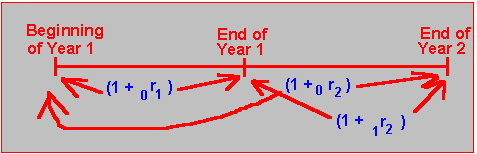In Figure 3.7, the two spot rates are:Now, compare two strategies:

1) Invest \$1 for two years.

2) Invest \$1 for one year and then reinvest it for another year.

In Strategy 1, you would get \$(1 + 0r2) at the end of the two years. In Strategy 2, you would have \$(1 + 0r1) at the end of one year, which you would have to reinvest at the one year interest rate at that time. When thought of today, this interest rate is called the forward rate that applies for the one year period starting at the end of Year 1. It is denoted as follows:It is computed by solving the equation: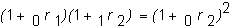This is simply the rate that equates the values of Strategies 1 and 2, given today’s interest rates.

From any yield curve, you can calculate a complete set of implied forward rates. In general, the implied forward interest rate between-period t and Period T, calculated today, solves the equation:Example 1

Assume the following 3 year term structure of spot interest rates:

 Rate 1+ Spot Rate 0r1 1.0400 0r2 1.0696 0r3 1.0989

From these spot rates, we can compute the implied set of spot and forward rates as follows:

 Rate Spot/Forward Rates 0r1 0.04 1r2 0.10 2r3 0.16

The spot rates in the first table are the geometric averages of the spot/forward rates in the second table. To verify this, consider the general equation where t = 1 and

T = 2:This yields:Or, by taking the square root of each side:Similarly, the three year spot rate is the geometric average of the one-, two-, and three year spot and forward rates:Online, you can use the software in the Bond Tutor titled "Yield Curve and Forward Rates" to change the yield curve and calculate all the forward rates and the values of all zero-coupon bonds. Note this is the subject after "Yield Curve and Term Structure."

First, in the smaller yield curve window, click on the button Numeric. This will pop up a small spreadsheet window that will allow you to directly enter the yield curve in spot rate form. For the present example, this results in: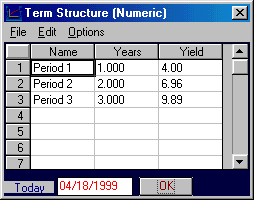Click on OK to update this yield curve. The yield curve window displays the new yield curve as follows:Now Bond Tutor calculates and graphs the forward interest rates for you. For the current problem these are:Clicking on the Numeric button on the Forward Rate window displays the forward rates numerically: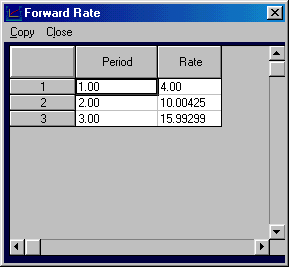Yield to Maturity, Forward Rates and Upward Sloping Yield Curves

We now return to the question we posed earlier, namely: Is the yield to maturity what you will actually earn?

Assume the spot and forward rates given in the example 1 above. You can verify that the price of a three year coupon bond with face a value equal to \$100 and a promised interest rate equal to 10% compounded annually is \$101.25. The yield to maturity from the coupon bond is computed numerically by solving for the interest rate r that equates the present value of the cash inflows to the bond’s price: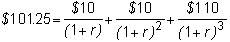The yield to maturity from acquiring this coupon bond for \$101.25 equals 9.502% per year.

To earn the yield to maturity over the life of the investment requires that investors reinvest their coupon payments at the yield to maturity. The problem, however, is that no such interest rate is available in the market. Instead, only spot interest rates are available. These spot rates, however, imply a set of forward rates over the periods of time that the coupon payments need to be reinvested. As a result, we can compare two courses of action:

1. Reinvest coupon payments at the yield to maturity.

2. Reinvest coupon payments at the forward interest rates implied from the spot rates.

The future value of Alternative 1 is.

 Timing of Cash Flow: End of Cash Flow Compounding Rate Future Value i. Year 1 \$ 10 1.09502^2 \$ 11.99 Year 2 \$ 10 1.09502 \$ 10.95 Year 3 \$110 1 \$110.00 Total \$132.94

Similarly, for Alternative 2, the future value is:

 Timing of Cash Flow: End of Cash Flow Compounding Rate Future Value ii. Year 1 \$ 10 1.10 x 1.16 \$ 12.76 Year 2 \$ 10 1.16 \$ 11.60 Year 3 \$110 1 \$110.00 Total \$134.36

You can see that the value is higher when you are reinvesting at the implied forward rates. This is because the upward sloping yield curve in the example means that the implied forward rates for Years 2 and 3 must be higher than in Year 1. Therefore, compounding at these rates results in more interest than does compounding at some weighted geometric average of all spot rates.

We can now return to the question we posed earlier: What are the interest rates at which coupons can be reinvested? The forward interest rates provide you information about the market’s expected future interest rates. Exactly how you interpret this information is the subject matter of Topic 3.10, Theories of the Term Structure of Interest Rates. For example, under the unbiased expectations theory, the forward interest rate gives you an unbiased estimate of the rate at which you expect to be able to reinvest your coupons.

We can make two observations about these forward rates. First, these rates are not guaranteed, because the yield curve itself may change. You will see later, however, that if you can trade forward contracts, you can guarantee (or "lock in") these rates in the future.

Second, even if the yield curve does not change over time, the yield to maturity is only applicable as an assumed reinvestment rate when the yield curve is horizontal. The yield to maturity has the advantage of simplicity for ranking returns from fixed-income securities. However, the yield to maturity is a hypothetical construct that, even with interest rate certainty, you cannot obtain in the marketplace for any security other than a zero-coupon bond.